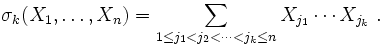## Monday, February 19, 2007

### Newton's Identities: Symmetric Polynomials

It is very easy to build an equation that has a certain set of solutions. If we wanted to build an equation where the solutions are 1,2,3,4,5 then all we need to do is multiply out the following:

(x - 1)(x - 2)(x - 3)(x -4)(x - 5)=0

If we carry out the multiplication, we can be sure that the result is an algebraic equation of power n=5 where we have the following:

ax5 + bx4 + cx3 + dx2 + e = 0

if x = 1, 2, 3, 4, or 5. We can figure out a,b,c,d,e by carrying out the multiplication.

Let's generalize this idea. Let's assume that the roots are r1, r2, ..., rn. Further, let's call each coefficient of the final result a1, a2, ..., an.

Then we have:

xn + a1xn-1 + ... + an-1x + an = (x - r1)*(x - r2)*...*(x - rn)

If xn has a coefficient, then we can divide the entire equation by a0 to get the above result.

We call a polynomial that results in this way, a symmetric polynomial. The idea is that we can switch any ri with any rj and it doesn't change the resulting polynomial.

Definition 1: Symmetric Polynomial

Let P(x1, x2, ..., xn) be a polynomial that consists of n variables. P(x1, x2, ..., xn) is said to be a symmetric polynomial if any two of these variables can be interchanged without changing the value of the polynomial.

Examples:

The following polynomials are symmetric.

P(x1, x2) = (x1)3 + (x2)3 - 7.

P(x1, x2) = 4x1x2

P(x1, x2, x3) = x1x2x3x2 - 2x1x3 - 2x2x3

The following polynomial is not:

P(x1,x2) = x1 - x2

Now, looking at the above equation again:

xn + a1xn-1 + ... + an-1x + an = (x - r1)*(x - r2)*...*(x - rn)

We can see that:

a1 = -r1 + -r2 + ... + -rn

We know this since there are n ways to get a value of xn-1. And they are:

-r1*x*x*x*...*x -r2*x*x*x*...*x - ... -rn*x*x*x*...*x

We can also see that an = (-r1)*(-r2)*(-r3)*...*(-rn)

since this is only way to multiply the values together to get x0. We can see that there exactly C(n,0)=1 ways to include x0. There are C(n,1)=n ways to to include only x1 and C(n,2) = ways to include x2, etc.

Indeed, in each case we can see that ai is the sum of C(n,i) different terms (see Lemma 3, here for details on C(n,r) if needed):

a2 = (-r1)*(-r2) + (-r1)*(-r3) + ... + (-rn-1)*(-rn) a3 = (-r1)*(-r2)*(-r3) + ... + (-rn-2)*(-rn-1)*(-rn) ... an-1 = (-r1)*...*(-rn-1) + (-r2)*...*(-rn)

Each of these terms is itself a polynomial. They are called the elementary symmetric polynomials σi.

Definition 2: Kth Elementary Symmetric Polynomials

A kth elementary symmetric polynomial is defined as:So, we have:

σk = (-1)kak where σk is called the kth elementary symmetric polynomial in r1, ..., rn

In my next blog, I will show that for every symmetric polynomial in r1, ..., rn can be expressed as an elementary symmetric polynomial in r1, ..., rn

References